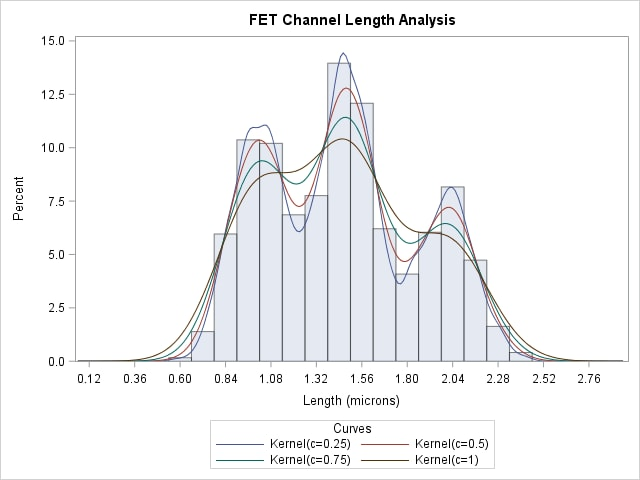# The UNIVARIATE Procedure

### Example 4.23 Computing Kernel Density Estimates

This example illustrates the use of kernel density estimates to visualize a nonnormal data distribution. This example uses the data set `Channel`, which is introduced in Example 4.15.

When you compute kernel density estimates, you should try several choices for the bandwidth parameter c because this determines the smoothness and closeness of the fit. You can specify a list of up to five C= values with the KERNEL option to request multiple density estimates, as shown in the following statements:

```title 'FET Channel Length Analysis';
proc univariate data=Channel noprint;
histogram Length / kernel(c = 0.25 0.50 0.75 1.00
l = 1 20 2 34
noprint)
odstitle = title;
run;

```

The L= secondary option specifies distinct line types for the curves (the L= values are paired with the C= values in the order listed). Output 4.23.1 demonstrates the effect of c. In general, larger values of c yield smoother density estimates, and smaller values yield estimates that more closely fit the data distribution.

Output 4.23.1: Multiple Kernel Density EstimatesOutput 4.23.1 reveals strong trimodality in the data, which is displayed with comparative histograms in Example 4.15.

A sample program for this example, uniex09.sas, is available in the SAS Sample Library for Base SAS software.